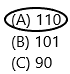Refer to our Texas Go Math Grade 1 Answer Key Pdf to score good marks in the exams. Test yourself by practicing the problems from Texas Go Math Grade 1 Module 10 Assessment Answer Key.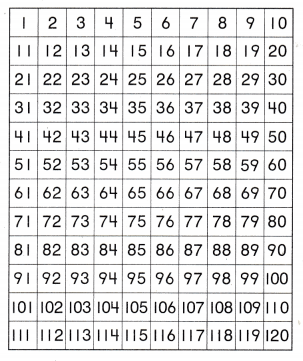Use the counting chart. Count backward by ones. Write the numbers. TEKS 1.5.A

Question 1.
52, 53, ___, ___, ___, ____
Answer: 52, 53, 54, 55, 56, 57.
It is a forward counting. Let’s take a look at the counting tracks. Notice the first row having 1 to 10. The second row has 11 to 20. Each number begin with 1, the second digit increases by 1 until the last column. In the third row, the pattern is the same only difference is the first digit is 2 instead of 1 and the second digit is increased by 1 until the last column. Notice that the second digit in each column is the same. Let’s skip down to 100 and the pattern continues. Now we have three-digit numbers like 101, 102, 103, 104, 105 and so on… and every third digit is increased by 1.Question 2.
115, 116, ____, ___, __, _____
Answer: 115, 116, 117, 118, 119, 120.
It is a forward counting. Let’s take a look at the counting tracks. Notice the first row having 1 to 10. The second row has 11 to 20. Each number begin with 1, the second digit increases by 1 until the last column. In the third row, the pattern is the same only difference is the first digit is 2 instead of 1 and the second digit is increased by 1 until the last column. Notice that the second digit in each column is the same. Let’s skip down to 100 and the pattern continues. Now we have three-digit numbers like 101, 102, 103, 104, 105 and so on… and every third digit is increased by 1.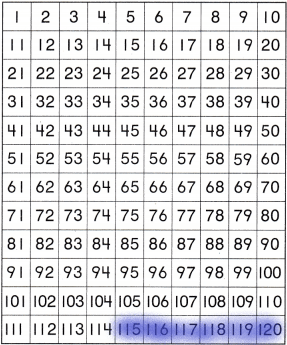Use the counting chart.
Count backward by ones.
Write the numbers. TEKS 1.5.A

Question 3.
74,73, ____, ___, __, _____
Answer: 74, 73, 72, 71, 70, 69.
Counting back requires us to count backwards. Backward counting is counting by removing one, every time. In backward counting, the numbers always are in descending order that means the numbers are from big to small.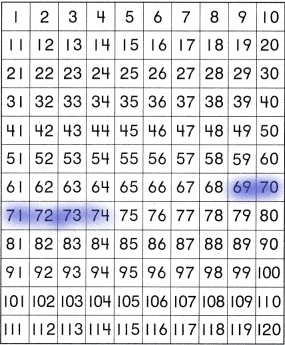Question 4.
112, 111, _______ , _______ , _______ , _______
Answer: 112, 111, 110, 109, 108, 107.
Counting back requires us to count backwards. Backward counting is counting by removing one, every time. In backward counting, the numbers always are in descending order that means the numbers are from big to small.Use the counting chart.
Count by tens.
Write the numbers. TEKS 1.5.C

Question 5.
60, 70, ____, ___, __, _____
Answer: 60, 70, 80, 90, 100, 110.
Explanation:
1. Skip counting in ten means to keep adding ten to a number.
2. A one hundred number grid is a useful way to teach skip counting.
3. Every time we add 10, we move one square down the number grid.
4. We count up in tens from 17, adding 10 each time.
5. We have 60, 70, 80, 90, 100, 110.
6. When skipping counting in tens, the numbers will all end in the same digit.
7. The digit in the tens column will increase by one each time.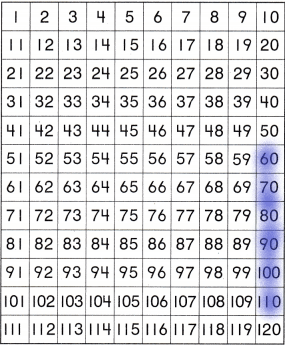Question 6.
55, 65, ____, ___, __, _____
Answer: 55, 65, 75, 85, 95, 105.
Explanation:
1. Skip counting in ten means to keep adding ten to a number.
2. A one hundred number grid is a useful way to teach skip counting.
3. Every time we add 10, we move one square down the number grid.
4. We count up in tens from 55, adding 10 each time.
5. We have 55, 65, 75, 85, 95, 105.
6. When skipping counting in tens, the numbers will all end in the same digit.
7. The digit in the tens column will increase by one each time.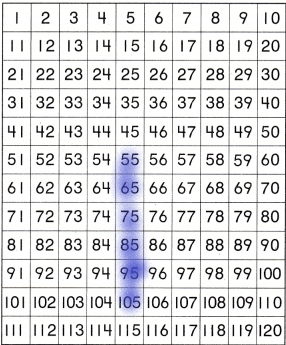Texas Test Prep

Question 7.
Which number begins the skip count by twos sequence? TEKS 1.5.B
(A) 4
(B) 2
(C) 1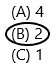Explanation:
The number begins with 2.
– Use number charts to skip count by 2s.
–  Skip count by 2s, given starting and ending numbers.
– Complete number patterns by filling in the missing numbers by counting by 2s.
– Count the number of objects arranged in pairs using skip counting.

Question 8.
Which number begins the skip count by fives sequence? TEKS 1.5.B
(A) 5
(B) 1
(C) 10Explanation:
– Count on and back by 5s within 1000 on a hundred chart.
– Fill in the missing values in a count-by-5s number sequence.
– Count the total number of objects in groups of 5 by skip counting by 5s.

Question 9.
Which number begins the skip count by tens sequence? TEKS 1.5.B
(A) 20
(B) 1
(C) 10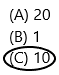Explanation:
Skip counting by 10 is the same as increasing the number of tens in a number. This involves counting on and counting back by 10’s, identifying counting patterns on a number chart( hundreds chart), continuing counting sequences and counting objects shown in groups of 10.

Question 10.
Which number is ten more than 100? TEKS 1.5.C
(A) 110
(B) 101
(C) 90